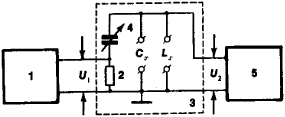# Quality Factor Meter

## Quality Factor Meter

(Q-meter), a radiometric instrument for determining the quality factor of oscillatory circuits, induction coils, and capacitors, as well as for measuring inductance, capacitance, ohmic losses in induction coils, and the loss-angle tangent of capacitors.

The quality factor of a circuit is defined as the ratio of voltage at resonance U2 to voltage U1 (see Figure 1); the scale of the device is calibrated according to the quality factor. The capacitance of a capacitor is determined by replacing it with a reference capacitor and connecting a reference induction coil to the terminals Lx of the circuit. To measure inductance, the coil is connected to the terminals Lx and the circuit C0Lx is tuned to resonance with the frequency of the oscillator; the inductance value is calculated according to Thompson’s formula: Lx = 1/(4π2 · f02 · C0), where Lx is the inductance being measured, f0 is the frequency of the oscillator, and C0 is the capacitance of the reference capacitor. The limits of measurement of the quality factor are 5-1,200 (at frequencies of 50 kilohertz to 250 megahertz); the error of measurement is 5-10 percent.Figure 1. Block diagram of a quality factor meter: (1) oscillator, (2) non-inductive resistor, (3) circuit system, (4) reference capacitor Cx. (5) electronic voltmeter

### REFERENCES

Tereshin, A. I., and V. A. Sofronov. Spravochnik po ekspluatatsii radioizmeritel’nykh priborov, 3rd ed. Kiev, 1969.
Valitov, R. A., and V. N. Sretenskii. Radiotekhnicheskie izmereniia. Moscow, 1970.

E. G. BILYK

Site: Follow: Share:
Open / Close